Presently sponsored by Serverless Guru: Your guide to cloud excellence, helping you every step of your serverless journey, including team training, pattern development, mass service migrations, architecting, and developing new solutions. Speak to a Guru today.

NOTE : read the rest of the series, or check out the source code.Fol­low­ing on from the last post, we will look at the Spreadsheet style today.

### Constraints

• The problem is modeled like a spreadsheet, with columns of data and formulas.
• Some data depends on other data according to formulas. When data changes, the dependent data also changes automatically.

Since we’re modelling the problem like a spreadsheet, let’s first define a few type alias to help us establish our domain language.

• a column is referenced by a string – e.g. column “A”, “B”, “C”, and so on
• a column can have explicit values, or a formula
• a formula references one or more other columns and uses their data to calculate the display value for the column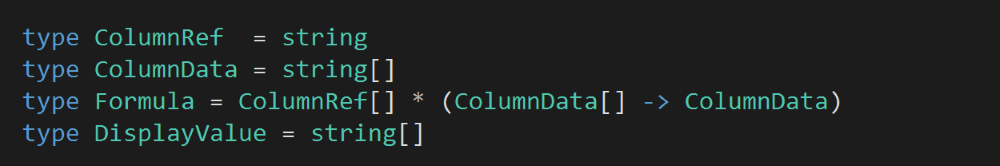We can model a Column with a union type, where a column is:

a) given explicit values; or

b) given a formula, and its display value is calculated from that formula.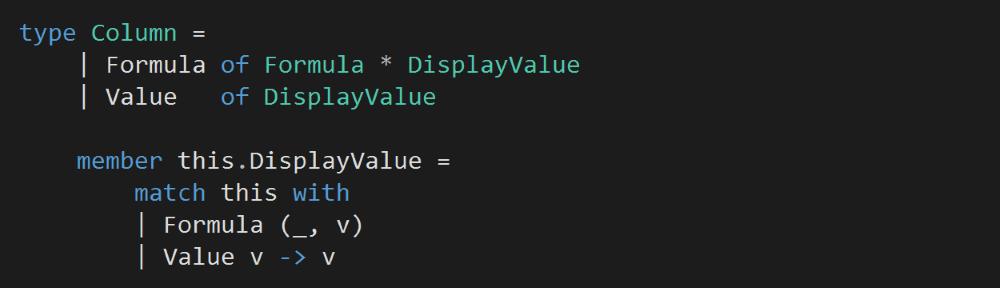A Spreadsheet is a collection of columns, whose value can be retrieved or updated using a ColumnRef, e.g.

spreadsheet.[“A”] <- Choice1Of2 [| “hello”; “world” |]

let columnA = spreadsheet.[“A”] // [| “hello”; “world” |]

Note that our custom indexer accepts a Choice<DisplayValue, Formula> in its setter instead of a Column. I made this decision because Column.Formula needs both the Formula and its calculated DisplayValue, and here it shouldn’t be the caller’s responsibility to exercise the formula and calculate its DisplayValue.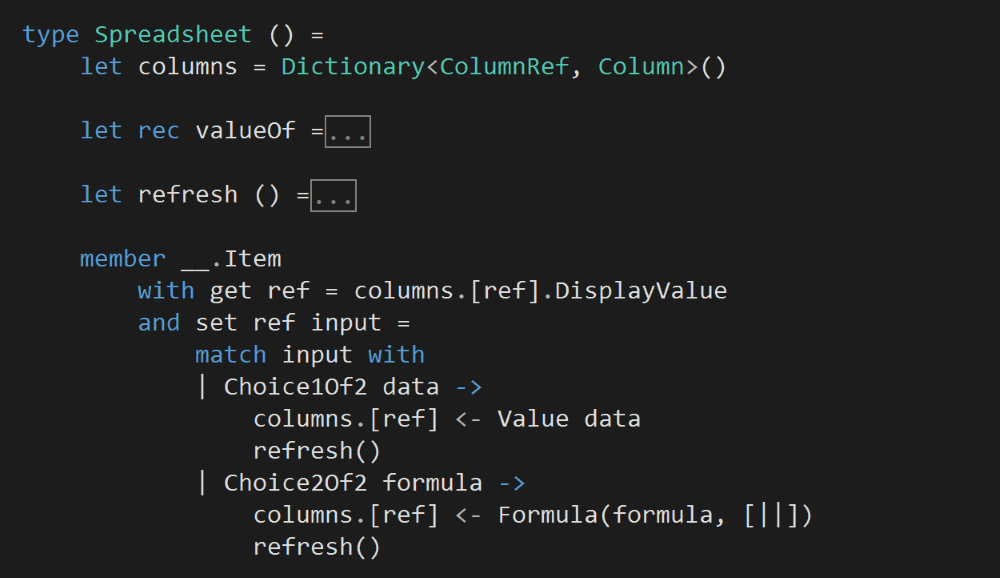After a column’s value is updated, we’ll recalculate the DisplayValue of all the columns in the Spreadsheet. This is a naive approach, but sufficient for the task at hand.

During this step, we’ll need to recursively evaluate the DisplayValue of the columns, sometimes a column might be evaluated multiple times if it’s referenced by several formulae. Again, there’s room for optimization here by caching previously calculated values.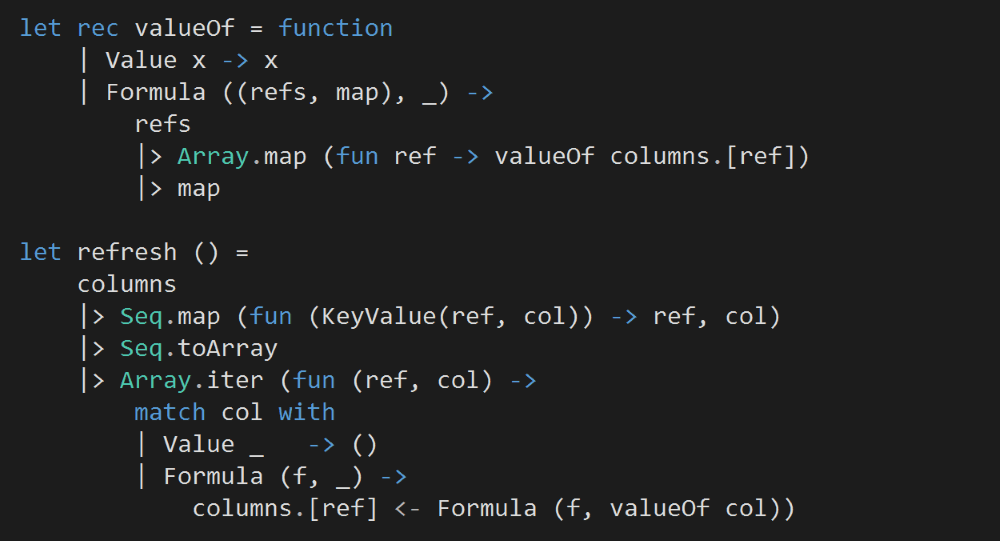Next, we’ll start inputting data into our Spreadsheet.

To make it easier to work with the Choice<DisplayValue, Formula> type, let’s add two helper functions.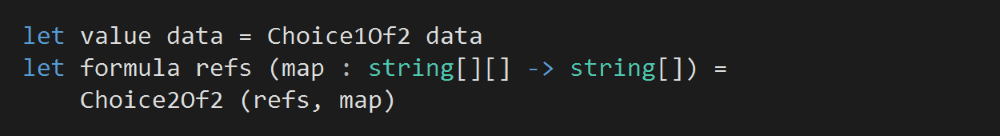We’ll leave column A and B blank for now.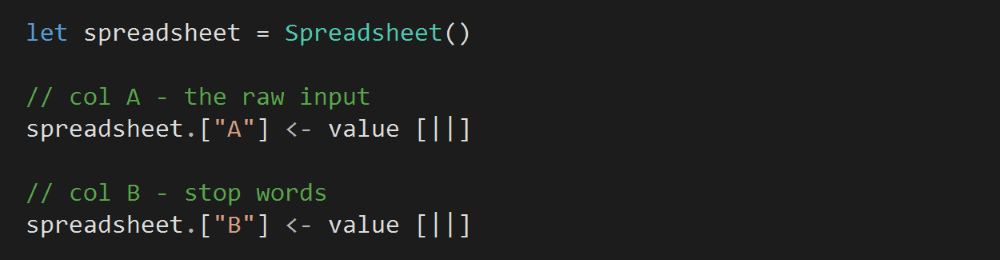Column C will use the data from Column A (all words) and Column B (stop words) to calculate all the non-stop words.

Column D will contain all the distinct words from Column C (non-stop words).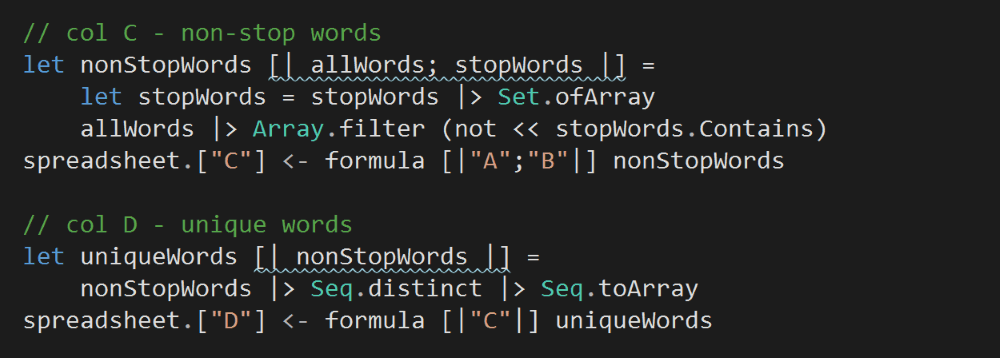Column E references both Column C (non-stop words) and Column D (distinct non-stop words), and counts the frequency for each of these words.

An important detail to note here is that, positionally, each count in Column E is aligned with the corresponding word in Column D.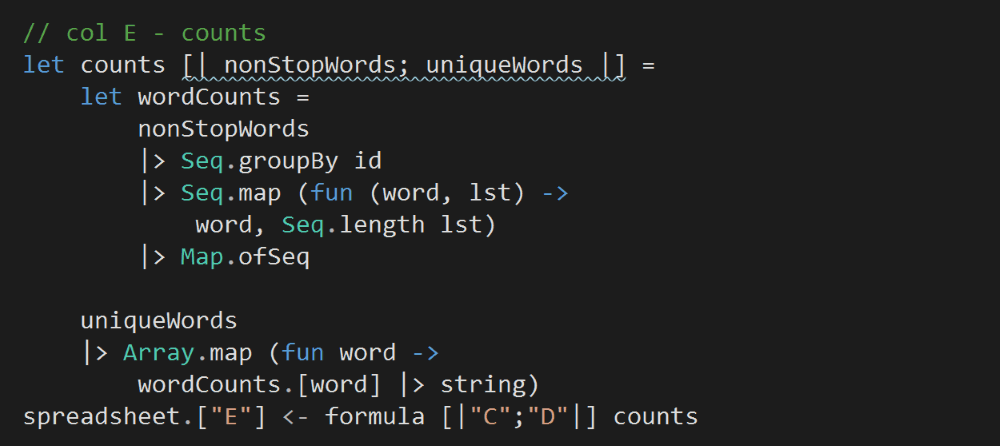Column F is where we’ll store the output of the program.

Because Column D (unique non-stop words) and Column E (counts) are positionally aligned, so we can use Array.zip to combine the two columns to calculate a sorted array of word frequencies.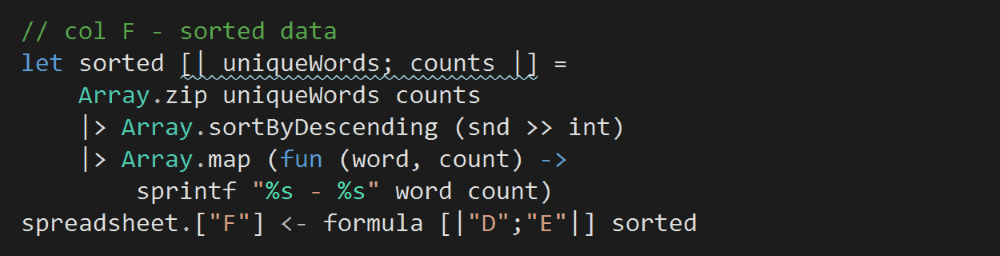Now that all the columns are set up, we can go back and input the text from Pride and Prejudice and the list of stop words into Column A and B respectively.

Doing so will trigger the DisplayValue of all the other columns to be recalculated.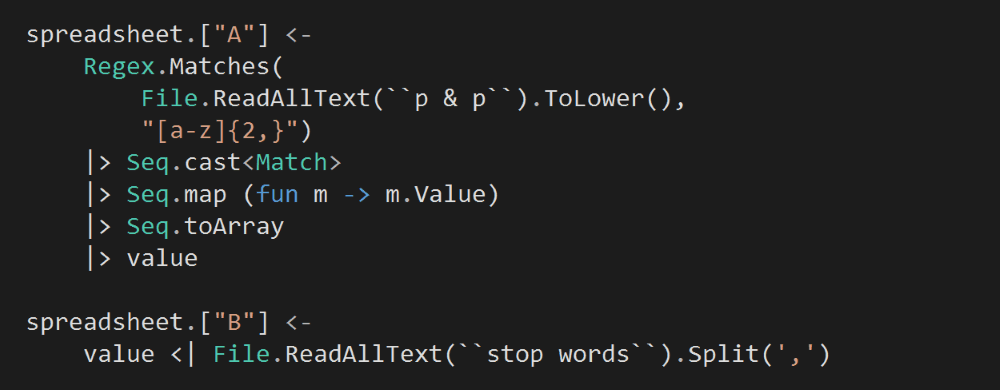Finally, we’ll take the top 25 rows from Column F and print them to complete our program.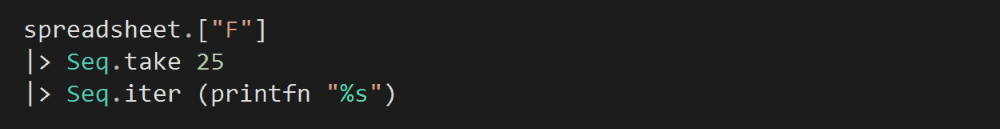I hope you’ve enjoyed today’s style, apologies for the lack of updates the last two weeks, I have been busy working out some stuff on a personal front. I’ll resume the two posts per week routine, which means we should finish this series in just over a month!

You can find the source code for this exer­cise here.